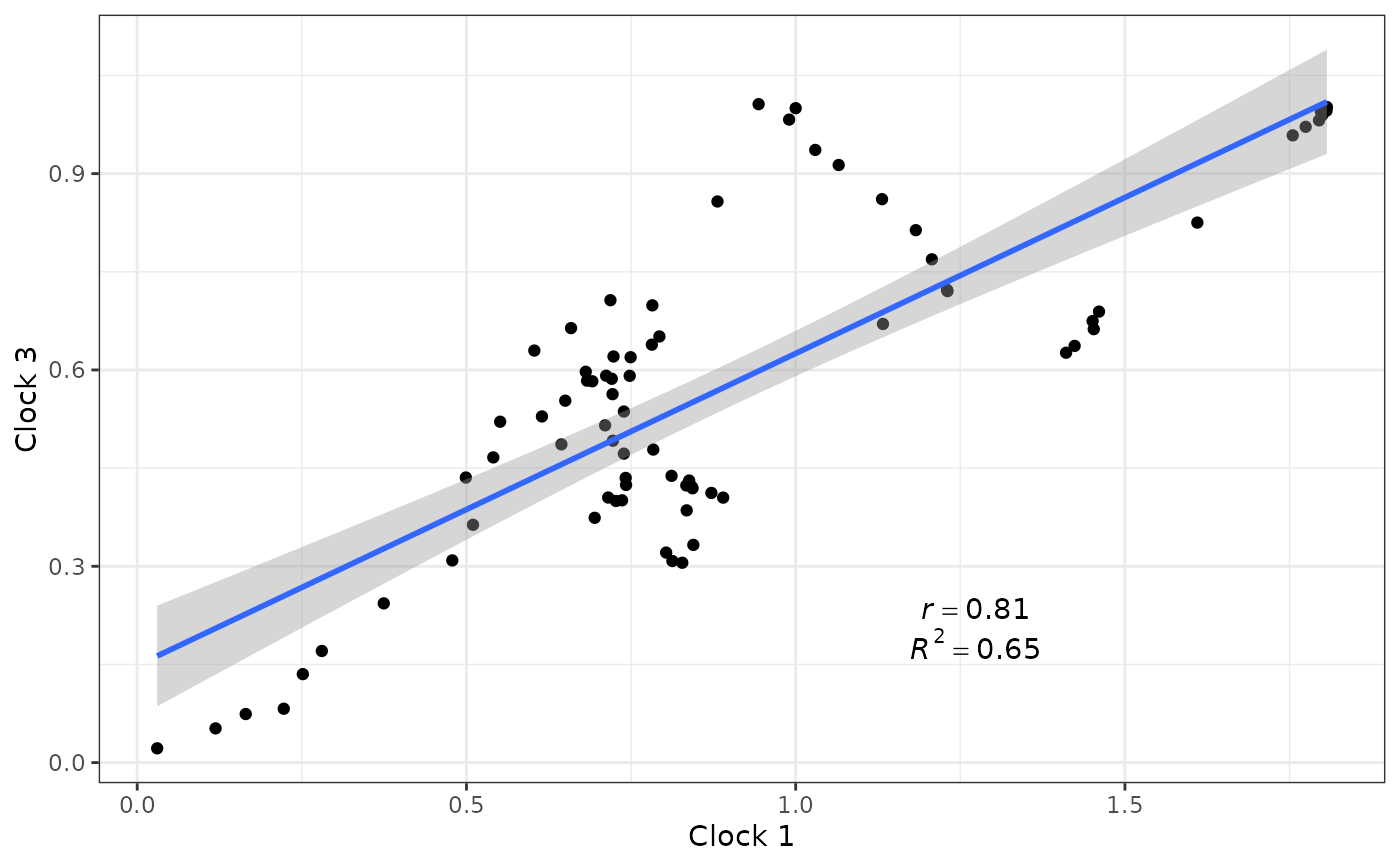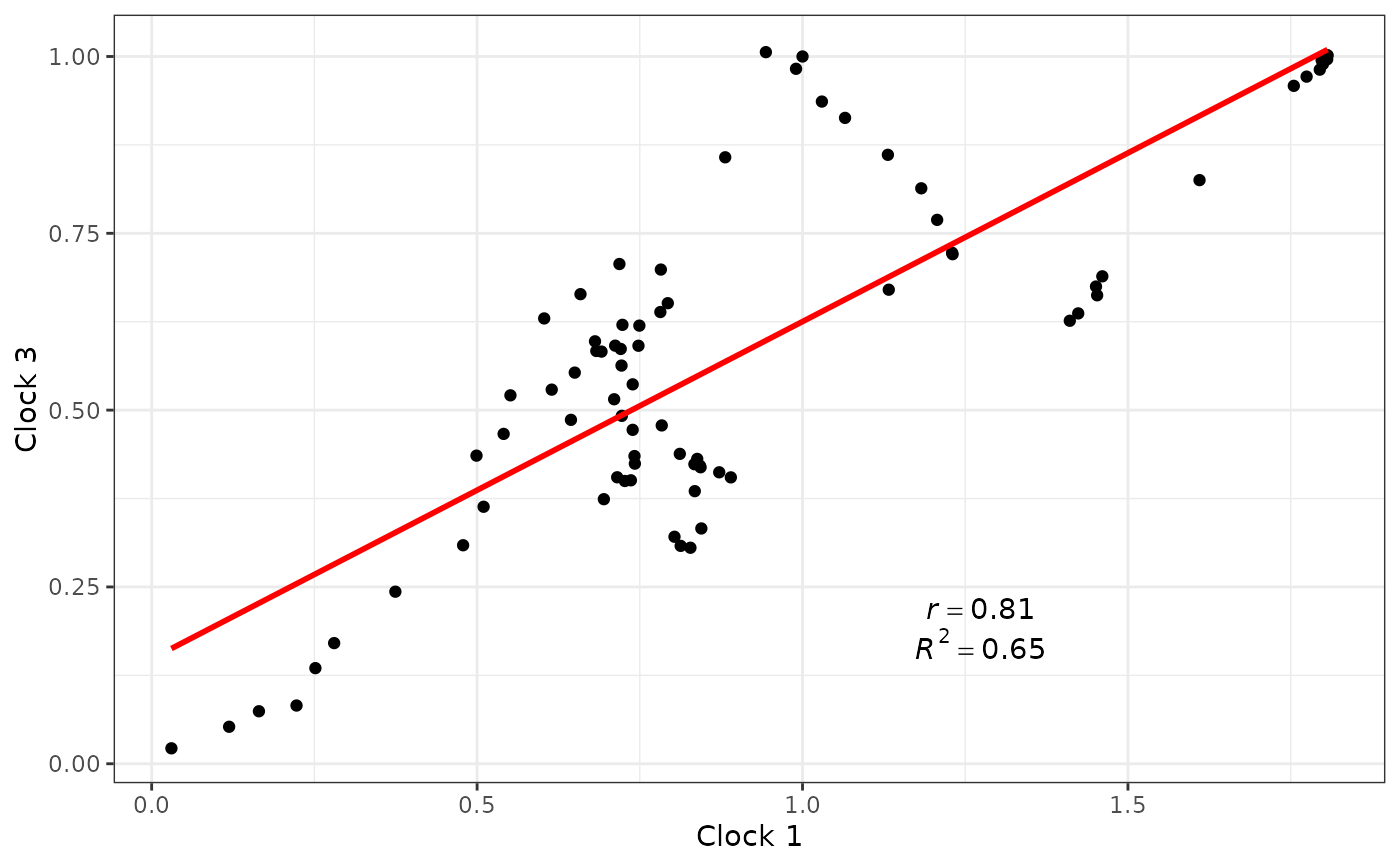Displays a scatterplot and fits regression line of one set of clock rates against another, optionally displaying their Pearson correlation coefficient (r) and R-squared values (R^2).

## Usage

clockrate_reg_plot(rate_table, clock_x, clock_y,
method = "lm", show_lm = TRUE,
...)

## Arguments

rate_table

A table of clock rates, such as from the output of get_clockrate_table_MrBayes.

clock_x, clock_y

The clock rates that should go on the x- and y-axes, respectively.

method

The method (function) used fit the regression of one clock on the other. Check the method argument in the to geom_smooth function of ggplot2 for all options. Default is "lm" for a linear regression model. "glm" and "loess" are alternative options.

show_lm

Whether to display the Pearson correlation coefficient (r) and R-squared values (R^2) between two sets of clock rates.

...

Other arguments passed to geom_smooth.

## Details

clockrate_reg_plot() can only be used when multiple clocks are present in the clock rate table. Unlike clockrate_summary and clockrate_dens_plot, no "clade" column is required.

## Value

A ggplot object, which can be modified using ggplot2 functions.

vignette("rates-selection") for the use of this function as part of an analysis pipeline.

geom_point, geom_smooth

## Examples

# See vignette("rates-selection") for how to use this
# function as part of an analysis pipeline